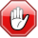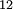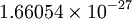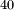# Atomic mass unitsThis page contains numerical values and/or equations. If you intend to use ANY of the numbers or equations found in SklogWiki in any way, you MUST take them from the original published article or book, and cite the relevant source accordingly.
The atomic mass unit (AMU) is defined as the twelfth part of the mass of a C$_{12}$ atom. Its value (in SI units, CODATA value) is$1.66054\times10^{-27}$ kilograms
Hence, it is approximately equal to the mass of a proton. Elements are assigned masses in these units which are not integer numbers. This is because neutrons and protons have slightly different masses, because nuclear binding forces cause the total mass of a nucleus to be less than that of the sum of its constituents, and, mainly, because of the appearance of natural isotopes. Thus, Potassium has a mass of 39.10 mainly because of natural K$^{40}$ isotopes.